Courses

# Error Detection & Encoding

## 10 Questions MCQ Test RRB JE for Computer Science Engineering | Error Detection & Encoding

Description
This mock test of Error Detection & Encoding for Computer Science Engineering (CSE) helps you for every Computer Science Engineering (CSE) entrance exam. This contains 10 Multiple Choice Questions for Computer Science Engineering (CSE) Error Detection & Encoding (mcq) to study with solutions a complete question bank. The solved questions answers in this Error Detection & Encoding quiz give you a good mix of easy questions and tough questions. Computer Science Engineering (CSE) students definitely take this Error Detection & Encoding exercise for a better result in the exam. You can find other Error Detection & Encoding extra questions, long questions & short questions for Computer Science Engineering (CSE) on EduRev as well by searching above.
QUESTION: 1

### In the 4B/5B encoding scheme, every 4 bits of data are encoded in a 5-bit codeword. It is required that the codewords haveat most 1 leading and at most 1 trailing zero. How many such codewords are possible?

Solution:

Answer is (C)
It says we have 5 bit codeword such that "it can't have two consecutive zeros in first and second bit" and
also" can't have two consecutive zeros in last two bits.
Code word with first two bits zero = 0|0|x|x|x| =8
Code word with last two bits zero = |x|x|x|0|0| =8
Code word with first and last two bits zero = 0|0|x|0|0| =2
Code word with first OR last two bits zero = 8+8-2=14
Therefore possible codewords =32-14 =18

QUESTION: 2

### Consider a 3-bit error detection and 1-bit error correction hamming code for 4-bit datq. The extra parity bits required would be ___ and the 3-bit error detection is possible because the code has a minimum distance of ____

Solution:

The Hamming distance between two bit strings is the number of bits that would have to flip to make the strings identical.
To detect d errors requires a minimum Hamming distance of d + 1 .
Correcting d bit flips requires a minimum Hamming distance of 2 * d + 1 , where d is number of bit in errors .
For the first blank , each error detection we need 1 parity bit
for 3 bit error detection we need 3 parity bit ...... So, 3 parity bit requires here. answer is 3.
Also we can calculate this way, formula is d+p+1 <= 2p where d=data bits , p = parity bits , d=4 bit given.
according to 1st question, d= 4 so 4+p+1<= 2p
p+5 <= 2p now if p=2 it becomes 7 <= 4 , Not satisfy . p = 3 it becomes 8 <= 8 , satisfy.
so p must be 3 .[ Minimum value of p is 3 ]
The second blank the 3-bit error detection is possible because the code has a minimum distance of ____ answer is
3+1=4, where d=3. Formula used d+1.
Answer for 2 blanks are [ 3,4 ]

QUESTION: 3

### In a communication network, a packet of length L bits takes link L1with a probability of P1 or link L2 with a probability of P2. Link L1 and L2 have bit error probability of b1 and b2 respectively. The probability that the packet will be received without error via either L1 or L2 is

Solution:

Probability of choosing link L1
= P1
Probability for no bit error (for any single bit) = 1 - b1
Similarly for link L2

Probability of no bit error = (1 - b2)
Packet can go either through link Lor  Lthey are mutually exclusive events (means one event happens other won't be
happening and so we can simply add their respective probabilities for the favorable case).
Probability packet will be received without any error = Probability of Lbeing chosen and no error in any of the L bits +
Probability of  L2 being chosen and no error in any of the L bits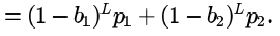Hence answer is option A .

Why option D is not correct choice here ?
Option D here is giving the probability of a frame being arrived with at least one bit correctly - i.., all the bits are not
errors.

QUESTION: 4

An error correcting code has the following code words: 00000000, 00001111, 01010101, 10101010, 11110000. What is themaximum number of bit errors that can be corrected?

Solution:

Answer: B
For correction: Floor of [(Hamming Distance - 1)/2] = Floor of [1.5] = 1 bit error.
For detection: Hamming Distance - 1 = 3 bit error.

QUESTION: 5

Data transmitted on a link uses the following 2D parity scheme for error detection:
Each sequence of 28 bits is arranged in a 4x7 matrix (rows rthrough r3, and columns d7 through d1 ) and is padded witha column dand row rof parity bits computed using the Even parity scheme. Each bit of column d0 (respectively, row r4 ) gives the parity of the corresponding row (respectively, column). These 40 bits are transmitted over the data link.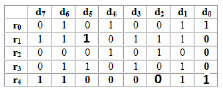The table shows data received by a receiver and has corrupted bits. What is the mini mum possible value of ?

Solution:Here we need t o change minmum 3 bits, so by doing it correct we get correct parity column wise and row wise (Correction
marked by dark number).
C is answer

QUESTION: 6

Let G(x) be the generator polynomial used for CRC checking. What is the condition that should be satisfied by G(x) to detect odd number of bits in error?

Solution:

Let me first explain building blocks to this problem. Before answering this, we should know the relationship between Sent
codeword, Received codeword, CRC generator and error polynomial.
let's take an example:
Sent codeword = 10010 (=x4+x)
Received codeword = 10110 (error at 2nd bit ) (=x4+x2+x)
GATE Overflow September 2017 161 of 740

Now, i can write Sent codeword = Received codeword + error (10010 = 10110 + 00100, here we do modulo 2
arithmetic i.e 1+1 =0 without carry )
in polynomial also we can see x4+x = x4+x2+x+x2 = x4+2x2+x = x4+x (here multiplier with 2 means 0 bcoz it
coressopnds to binary modulo 2 arithmetic which is 1+1 = 0 (not 2) )

or

We can also write Received codeword = Sent codeword + error (Check it using same mrthod as above)
Sent codeword C(x), Received codeword R(x) and error E(x).
Now we have R(x) = C(x) + E(x). and let CRC polynomial be G(x). G(x) always divides C(x), and if there is an error then
G(x) should not divide R(x). Lets check -
R(x) mod G(x) =(C(x) + E(x)) mod G(x) (for simplicity i am writing mod as division )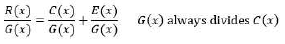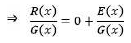If G(x) divides E(x) also this would mean G(x) divides R(x). We know that, If G(x) does not properly divide R(x) then there
is an error but we are never sure if there is error or not when G(x) divides R(X).
As we saw, G(x) divides R(x) or not totally depends on G(x) divides E(x) or not. whole strength of G(x) lies if it does not
divide any possible E(x).
Lets see again E(x). if there is an error in 3rd and 4th bit from left (LSb is 0th bit ) then E(X) = x4+x3.( it does not matter
error is from toggling 1 to 0 or 0 to 1) Check with above example.
Now come to question. it says G(x) should detect odd number of bits in error?. If number of bits are odd then terms in E(x)
would be odd.
for instance if 1st, 2nd and 5th bit got corrupted then E(x) = x5+x2+x.
It is clear that if any function f(x) has a factor of x-k, then at x=k, f(x) would be zero. I.e.f(x) = 0 at x=k.

• We want to detect odd number of bits that means received message R(x) contains an odd number of inverted bits, then

E(x) must contain an odd number of terms with coefficients equal to 1.

• As a result, E(1) must equal to 1 (remeber 1+1 = 0, 1+1+1 = 1. Any Odd number of times sum of one's is = 1). E(1) is not zero, this means x+1 is not a factor of E(x).
• Now I want G(x) not to be a factor of E(x), So that G(x) wont divide E(x) and i would happily detect odd number of bits.
• So, if we make sure that G(1) = 0, we can conclude that G(x) does not divide any E(x) corresponding to an odd number of error bits. In this case, a CRC based on G(x) will detect any odd number of errors.
• As long as 1+x is a factor of G(x), G(x) can never divide E(x). Because we know E(x) dont have factor of 1+x .

Option C.

QUESTION: 7

A layer-4 firewall (a device that can look at all protocol headers up to the transport layer) CANNOT

Solution:

Answer is (D).
(A) It is POSSIBLE to block "entire" HTTP traffic by blocking all the traffic on port number 80 Since here we DON'T need to check anything that is application layer specific. We only need to block port no 80 for
required time span.
(B) & (C) are fairly possible to achieve.
(D) However (D) is not possible to achieve although the service uses TCP at transport layer. But see the question. We dont need to block entire TCP traffic so we cant block any specific PORT number. Also it is given
that IT IS MULTI- USER System and so many user may be using same port for communication. Therefore blocking that port would block all the users WHILE we want a specific user. So how to do that. To do so we
need Application layer specific information of the user like user_id type of things which cant be checked as it is 4-layer firewall.
So it is not possible to allow other users and block some specific at the same time using a 4-layer firewall (unless they all be using different port numbers which we actually cant predict).

QUESTION: 8

Following 7 bit single error correcting hamming coded message is received.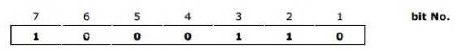Determine if the message is correct (assuming that at most 1 bit could be corrupted). If the message contains an error find the bit which is erroneous and gives correct message.

Solution:

There is error in This message. Error is in bit 6.

How to calculate it ? First of all reverse given input to get it in correct position from 1 to 7.

0110001

Bit 1, Bit 2 & Bit 4 are partity bits.

Calculating position of error =>

c3 c2 c1

1 1 0

Here c1 = bit4⊕bit5⊕bit6⊕bit7=0⊕0⊕0⊕1=1 (Taking Even parity )

c2 = bit2⊕bit3⊕bit6⊕bit7=1⊕1⊕0⊕1=1

c3 = bit1⊕bit3⊕bit5⊕bit7=0⊕1⊕0⊕1=0

Hamming%287,4%29

When you correct bit 6 .

You get message as 0110011.

IF you calculate C1,c2,c3 all will be 0 now !

QUESTION: 9

A message is made up entirely of characters from the set x = {P,Q,R,S,T} . The table of probabilities for each of the  characters is shown below: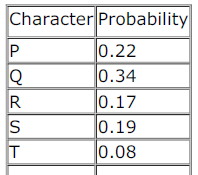Total                       1.00
If a message of 100 characters over X is encoded using Huffman coding, then the expected length of the encoded message
in bits is ______.

Solution: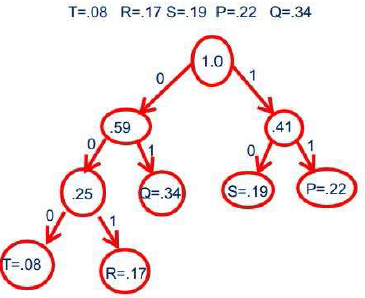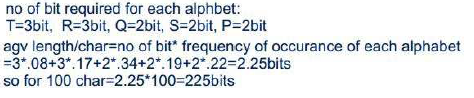QUESTION: 10

What is the distance of the following code 000000, 010101, 000111, 011001, 111111?

Solution:

Distance (also called min-distance) of a block code is the minimum number of positions in which any two distinct codes
differ. Here, min-distance occurs for the codes 2 and 3 and they differ only in 2 positions. So, d = 2.